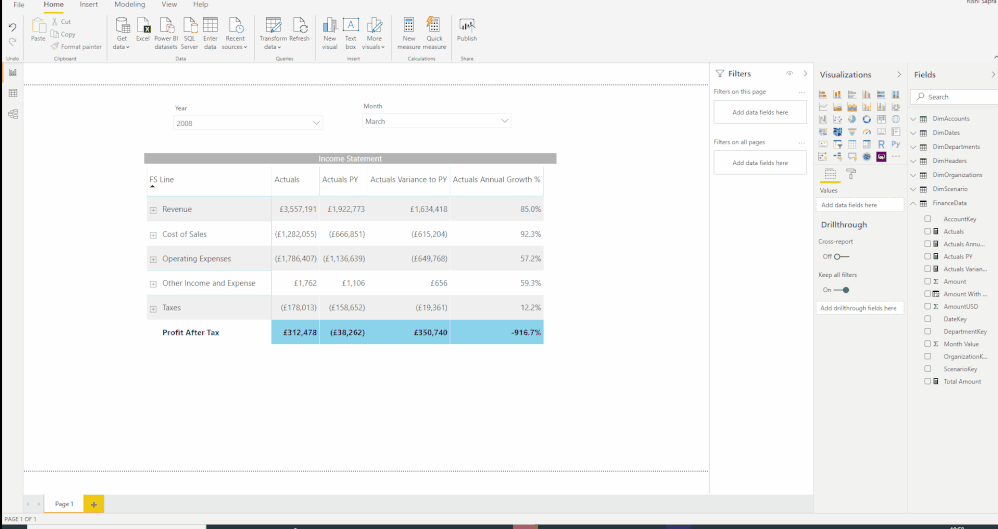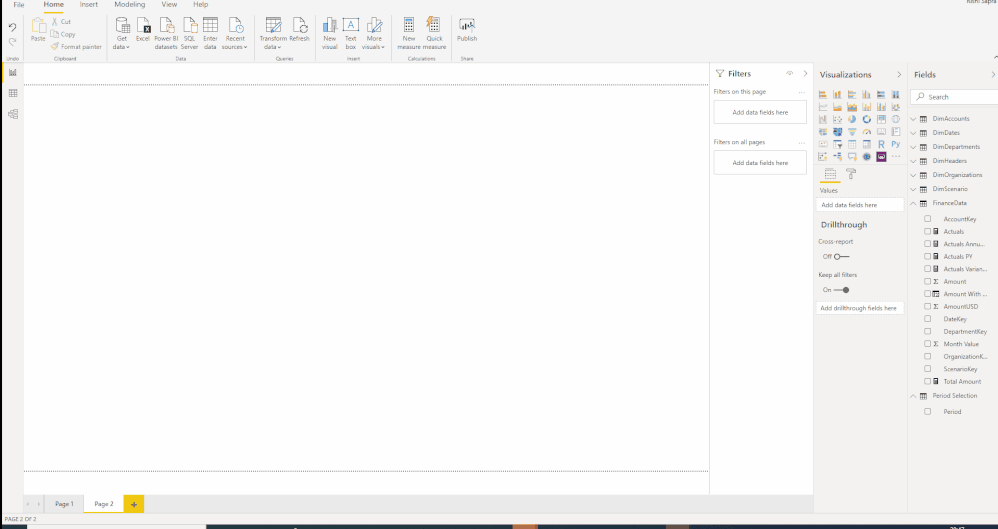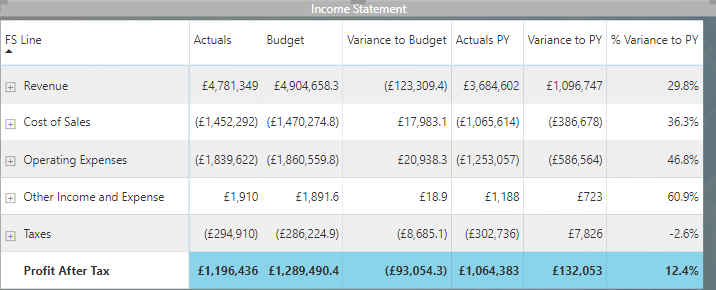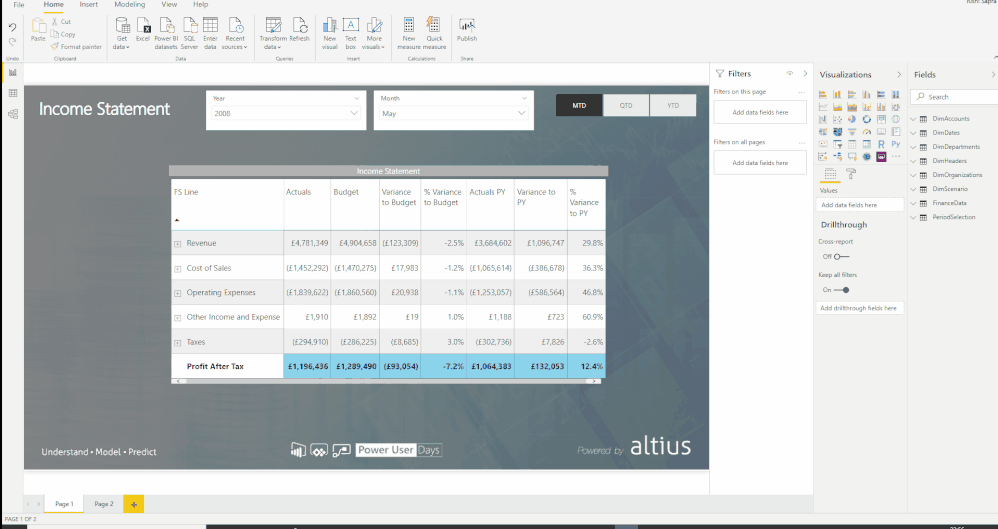cancel
Showing results for
Did you mean:## Income Statement Analysis: Part 3 of 5: Format the page and add Dynamic Measure

In the previous posts (part 1 and part 2), we set up a matrix visual which shows our total actuals, Prior Year Actuals and Variance to Prior Year for a given year/month (based on the slicer selection).

In this section we want to take this a step further and choose to show either Monthly figures, or alternatively Quarter to Date or Year to Date for the selected year and month.

We will also apply a background and theme to the report and will apply conditional formatting to the income statement matrix, dynamically calculating whether an increase in the number is good or bad (i.e. whether it is a revenue/profit or expense number).

# 1         Create Parameter Table/Slicer for Period Selection

The first thing we want to have is a slicer with the values MTD, QTD and YTD; when the user selects a value the income statement should update to reflect the monthly/quarterly/year to date values appropriate.

Slicers need to be populated with a list of values from a field in a table though we don’t currently have a field with these values, so we’ll have to create one:

Click on ‘Enter Data’ in the Home tab of the ribbon and create a new table called Period Selection with a single column of the same name with 3 values – ‘MTD’, ‘QTD’ and ‘YTD’ then click load:This has now created a new table in the data model. However, unlike the others this one won’t have relationships with any other tables – there is no field on which to create a relationship. Instead we leave it as a disconnected table; we will pick out the value selected by the user and use this as part of our logic for the measures shown in the Income Statement.

Even though this table has no relationships, we can still add the field as a slicer on the page with the following formatting options.

• General: Orientation as Horizontal
• Selection Controls: Single Select On
• Title: Off# 2         Create Dynamic Actuals Measure based on period selection

Now we need a measure which is going to calculate the Actuals value depending on the period selection chosen in the slicer.

Create a new measure in the FinanceData table called ‘Total Actuals By Period’:

``````Actuals By Period =

VAR SelectedPeriod = SELECTEDVALUE(PeriodSelection[PeriodSelection])
RETURN
SWITCH(
SelectedPeriod,
"MTD",
CALCULATE([Actuals],DATESMTD(DimDates[Date])),
"QTD",
CALCULATE([Actuals],DATESQTD(DimDates[Date])),
"YTD",
CALCULATE([Actuals],DATESYTD(DimDates[Date])),
[Actuals]
)``````

This measure starts by picking out the value selected in the slicer (i.e. the field) - there will always be one value selected since we enabled single select on the slicer. The SWITCH function then returns different results on depending on the value selected. For example, if MTD is selected, then the expression

``    CALCULATE([Actuals],DATESMTD(DimDates[Date]))``

will be evaluated. The function DATESQTD and DATESYTD is used when these periods are selected respectively. These are all time intelligence functions which are made possible by us ingesting a date table and marking it as such in Step 1.

Once we have created the new measure, we need to update the number format. Navigate to the relationships view window (the third one in the left panel), selecting “Actuals By Period”, choose format “Custom” and enter in the “Custom Format”: "£"#,0;("£"#,0);"£"#,0.  This will show numbers with a pound sign, a comma separator and negative numbers in brackets:We will now use this measure instead of the Actuals one in our income statement. Replace the Actuals value in the Income Statement matrix with the new Actuals By Period measure (Changing the display name back to Actuals). Check that the numbers now show correctly depending on whether MTD/QTD/YTD is selected.

We also need to update the Actuals PY and Actuals Variance to PY Measure to refer to this new measure instead:# 3         Create Budget Measures

If we want to show a comparison between Actuals and Budget in our Income statement (in addition to the comparison to PY), then we can create the following measures in exactly the same way as we did for Actuals:

``Budget = CALCULATE([Total Amount],DimScenario[Scenario]="Budget")``

``````Budget By Period =
VAR SelectedPeriod = SELECTEDVALUE(PeriodSelection[PeriodSelection])
RETURN
SWITCH(
SelectedPeriod,
"MTD",
CALCULATE([Budget],DATESMTD(DimDates[Date])),
"QTD",
CALCULATE([Budget],DATESQTD(DimDates[Date])),
"YTD",
CALCULATE([Budget],DATESYTD(DimDates[Date])),
[Budget]
)``````

``Variance to Budget = [Actuals By Period] - [Budget By Period]``

``% Variance to Budget = DIVIDE([Variance to Budget],[Budget By Period])``

Then drag the new measures into the Income statement matrix and rename the display names so that it now looks like:# 4         Apply Background/Theme

Click on the Formatting pane for the page (With no visual selected), under Page Background click on ‘Add Image’ and navigate to Background2.png in the Assets folder under the zip folder contents extracted in Step 1.  Change the Transparency to 0%.

Under the View tab of the ribbon, navigate to the Themes section, Browse for theme and Import the theme PowerUserDays.json in the same assets folder:Rename the page to ‘Income Statement’.

For the year and month slicers, set the background to white with 0% transparency.

Add a text box with the text ‘Income Statement’, with a white font colour of size 24 & turn off the background. Place the elements on the page so that it now looks like:# 5         Apply Conditional Formatting to the Income Statement

Next, we want to apply conditional formatting to the Income Statement variance columns, highlighting good variances (increase in revenue/profit or decreases in cost) in Green and bad variances in Red.

For this we need to add measures to distinguish whether the variance to Budget/PY is good or bad and return a green or red colour accordingly:

``````Positive Change Good or Bad-IS Number to Budget =
VAR GoodColour = "#00B7C3"
VAR Sign = SELECTEDVALUE(DimAccounts[Sign])
VAR IsNegativeLine = Sign=-1
VAR Delta = [Variance to Budget]
VAR DeltaIsPositive = Delta >0
VAR IsChangegood = SWITCH(TRUE(),
AND(DeltaIsPositive,IsNegativeLine),"Good",
AND(DeltaIsPositive,NOT(IsNegativeLine)),"Good",
RETURN

``````Positive Change Good or Bad-IS Number to PY =
VAR GoodColour = "#00B7C3"
VAR Sign = SELECTEDVALUE(DimAccounts[Sign])
VAR IsNegativeLine = Sign=-1
VAR Delta = [Actuals Variance to PY]
VAR DeltaIsPositive = Delta >0
VAR IsChangegood = SWITCH(TRUE(),
AND(DeltaIsPositive,IsNegativeLine),"Good",
AND(DeltaIsPositive,NOT(IsNegativeLine)),"Good",
RETURN

These measures start off defining a red colour as bad and a green colour as good, and then uses the sign field to determine whether an increase is good or bad returning the corresponding colour. The SWITCH function is used again but the condition being evaluated is simply TRUE(), so it returns the result (“Good” or “Bad”) on the first condition that is met.

We can then use this measure to apply conditional formatting to the variance columns on the matrix. On the formatting tab of the matrix, we expand the conditional formatting section, select a variance column in the drop-down (e.g. Variance to Budget), turn on ‘Background Color’ and click on ‘Advanced Controls’. In the conditional formatting dialog that comes up we are presented with 3 options for applying advanced conditional formatting:

• Colour Scale: Every number in the variance column is assessed relative to all the other numbers in the column with the lowest/highest value being assigned the colour that is set for these, and all other numbers have a colour relative to the colour gradient scale that is set here

• Rules: The colours are set based on rules/thresholds, based either on the variance measure itself or another field/measure. For example, we could set all variances above £20k to Red, those between £5k and £20k as Orange, and those less than £5k as Green. Alternatively, we could define a measure that is somehow based on the variance measure which returns discrete values (e.g. 1 or 0) and use this as the basis for the rules.

• Field Value: This can be used when we have a field/measure which contains HEX colour codes (e.g. ‘#FFFF’). This is a useful way of explicitly assigning colours to dimension values rather than manually assigning them in the formatting pane of each visual. Having the corresponding colour codes for dimension values (in this case it might be a colour assigned to e.g. each organisational unit) means that those colours will be consistent across visuals and you can colour values even if they aren’t present in the visual at the time of building.

In our scenario, we could have defined measures which return “Good” or “Bad” (or e.g. 1 or 0) for the variance, and then used Rules to set the colour accordingly. In this case, however, we returned the HEX colour codes directly in the measures so we can use the Field Value type in order to set the colours:If this same conditional formatting rule is applying to the Variance to Budget and PY columns, the resulting Income statement should now look like:The report should now look like the PBIX attached.